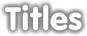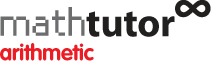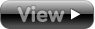Video tutorials, diagnostics, text and interactive exercises covering over 80 maths topics.## Arithmetic

• Fractions Basic Ideas
• Fractions Multiplying And Dividing
• Decimals
• Percentages
• Ratios
• Rules Of Arithmetic
• Surds
•## Algebra

• Mathematical Language
• Powers or indices
• Logarithms
• Substitution And Formulae
• Expanding And Removing Brackets
• Pascal's triangle and the binomial theorem
• Transposition of Formulae
• Linear Equations in one variable
• Completing The Square
• Completing The Square Maxima And Minima
• Simultaneous Linear Equations
• Solving Inequalities
• Cubic Equations
• Simplifying Algebraic Fractions
• Polynomial Division
• Partial Fractions
•## Functions, Graphs, Sequences & Series

• Introduction to functions
• Linear functions
• Polynomial functions
• Exponential and logarithm functions
• Trigonometric functions
• Hyperbolic functions
• Composition of functions
• Inverse functions
• Sigma notation
• Arithmetic and geometric progressions
• Limits of sequences
• The sum of an infinite series
• Limits of functions
•## Geometry & Vectors

• Properties of straight line segments
• The gradient of a straight line segment
• Equations of straight lines
• The geometry of a circle
• Conic sections
• Polar co-ordinates
• Introduction to vectors
• Cartesian components of vectors
• The scalar product
• The vector product
•## Trigonometry

• Pythagoras' Theorem
• Trig ratios in a right-angled triangle
• Trig ratios of an angle of any size
• Trig equations
• Trig identities
• Triangle formulae
• Cosec, sec and cot
• The double-angle formulae
• a cos x + b sin x = R cos (x - a)
•## Differentiation

• Differentiation from first principles
• Differentiating powers of x
• Differentiating sines and cosines
• Differentiating logs and exponentials
• Using a table of derivatives
• The quotient rule
• The product rule
• The chain rule
• Parametric differentiation
• Differentiation by taking logarithms
• Implicit differentiation
• Extending the table of derivatives
• Tangents and normals
• Maxima and minima
•## Integration

• Integration as summation
• Integration as the reverse of differentiation
• Integration using a table of anti-derivatives
• Integration by parts
• Integration by substitution
• Integrating algebraic fractions (1)
• Integrating algebraic fractions (2)
• Integration using trigonometric formulae
• Finding areas by integration
• Volumes of solids of revolution
• Integration leading to log functions